# Economic Analysis of Energy Consumption Based on Thermoeconomic Cost Analysis Model

Economic Analysis of Energy Consumption Based on Thermoeconomic Cost Analysis Model

Weitao Wu

Department of National Defense Economy, Army Logistics University of PLA, Chongqing 401331, China

Corresponding Author Email:
291688472@qq.com
Page:
620-624
|
DOI:
https://doi.org/10.18280/ijht.370233
5 December 2018
|
Accepted:
10 April 2019
|
Published:
30 June 2019
| Citation

OPEN ACCESS

Abstract:

With the deepening of industrialization, energy resources are of strategic importance to China, as they lay the foundation for social and economic development. The energy consumption pushes up the economic cost of the industry, but the enterprises can obtain lots of benefits by utilizing the residual heat. This paper adopts the thermoeconomic cost analysis model, which examines economic factors through thermodynamic analysis, to disclose the relationship between energy consumption and economic growth in China. In addition, the energy consumption was optimized through a case study on iron and steel enterprises, in light of thermoeconomics. The results show that China’s economic growth has linear and nonlinear dynamic correlations with the total energy consumption, coal consumption and oil consumption; the total energy consumption grows at a slower rate than economy, and the energy consumption rate exhibits a declining trend; residual heat recovery and utilization can greatly reduce the level and rate of energy consumption, and enhance the output energy value, thus lowering the thermoeconomic cost. The research findings lay the theoretical basis for the application of thermoeconomic cost in energy consumption analysis.

Keywords:

energy consumption, residual heat utilization, thermoeconomic cost, thermodynamics, iron and steel enterprises

1. Introduction

An energy resource is a material that produces the energy needed to satisfy our production and living needs. Energy resources are of strategic importance to China, as they lay the foundation for social and economic development . The consumption of energy resources, i.e. energy consumption, is critical to world economic growth. In fact, the global economic boom in recent years is driven by the continuous growth in energy consumption.

Energy consumption, as a kind of thermodynamic cost, can be analyzed by thermoeconomics. The way to analyze thermodynamic cost by economic tools is known as the thermoeconomics on energy consumption. This analysis approach often treats the material, energy and cash circulating in the heat consumption system as heat energies. In general, there are two types of equipment in the heat consumption system, namely, generation equipment and dissipation equipment [2-4]. The former includes boilers, steam turbines and generators in power plants, while the latter includes condensers, induced draft fans and dust removal devices .

Many scholars have noted the correlation between energy consumption and economic growth. However, there is not yet a unified understanding about the correlation. With the continued increase in energy consumption across China, it is far from enough to examine the relationship between the total energy consumption and economic growth . Considering the dependence of energy consumption on income level, energy price, urbanization level and industrial structure, the trend of economic growth must be modelled under the constraint of limited energy, before achieving sustainable development of the economy [7-8]. Some scholars discovered that positive correlation exists between energy consumption and economic cost, and between energy consumption rate and economic growth, both of which naturally lead to the positive correlation between thermoeconomic cost and economic growth .

In this paper, the thermoeconomic cost analysis model is adopted to discuss the relationship between energy consumption and economic growth in China. On this basis, the energy consumption of iron and steel enterprises was optimized by thermoeconomics.

2. Energy Consumption in China and Thermoeconomic Cost Analysis Model

2.1 Energy consumption in China

China now takes an increasingly large share in global energy consumption. The country has become the second largest consumer of primary energy in the world. The energy consumption in China is greatly affected by the changing industrial structure . Coal, oil, natural gas and electricity are the main energies in China’s energy consumption structure . The association between energy and economic growth is mainly reflected by the immense economic contribution of natural resources. Some scholars have found the long-term cointegration between the total energy consumption, coal consumption and GDP in China . The total energy consumption measures the overall consumption of energy in a country within a period. It equals to sum of all energies consumed in material production sector, non-material production sector and the field of life .

Currently, China manages to maintain fast economic growth, despite the slow increase in energy consumption . The total energy consumption and its contribution to GDP is illustrated in Figure 1. It can be seen that the total energy consumption increases linearly with the elapse of time, while its contribution to GDP grows at a different pace.

## 1.png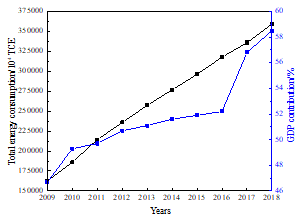Figure 1. Time variations of total energy consumption and its contribution to GDP

2.2 Thermoeconomic cost analysis model

Thermoeconomics is a cross-discipline of thermodynamics and economics. In thermal economy, the energy is converted from a physical quantity to economic dimension, which facilitates the energy generation and consumption. The thermoeconomic cost is usually analyzed in matrix mode. In the narrow sense, thermoeconomic cost specifically refers to the energy or fuel being consumed. In the broad sense, thermoeconomic cost also covers the equipment depreciation, operational cost and management cost. Overall, thermoeconomic cost can be described as:

crEp=cnEn+Cn  (1)

$\mathrm{C}_{\mathrm{n}}=\sum_{1}^{n} Z_{i}+\mathrm{R}$  (2)

where Ep and En are the energy values entering and leaving the system, respectively; cr and cp are the unit prices of the energy entering and leaving the system, respectively; Cn is the non-energy cost for the system to maintain normal operation; Zi is the equipment depreciation; R is the operational cost and management cost of the system.

## 2.pngFigure 2. The relationship between energy load and thermal efficiency

Taking diesel engines as an example, the thermoeconomic cost mainly occurs during exhaust ventilation and cooling. According to the energy load-thermal efficiency curve in Figure 2, the thermal efficiency first increases and then decreases with the growth of energy load, and peaks at the energy load of 75 %. Hence, the thermoeconomic cost is minimized at this energy load. Figure 3 presents the relationship between energy load and energy consumption rate. It can be seen that, with the growth in energy load, the main engine consumes less energy in exhaust ventilation, while more energy is consumed in air cooling and jacket cooling.

## 3.png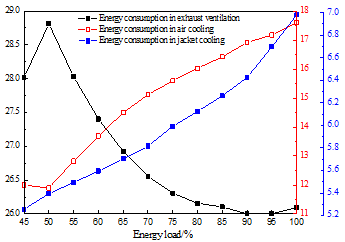Figure 3. The relationship between energy load and energy consumption

3. Analysis on the Relationship Between Energy Consumption and Economic Growth

3.1 Linear dynamic relationship between energy consumption and economic growth

The relationship between energy consumption and economic growth is essentially how GDP correlates with the total energy consumption, coal consumption and oil consumption. In previous studies, GDP and the three factors were converted to logarithmic forms, and prepared into time series plots. These plots demonstrate the consistency between energy consumption and economic growth. However, the total energy consumption grows at a slower rate than economy, and the energy consumption rate exhibits a declining trend.

The vector error correction model was introduced to describe the long-term cointegration between GDP and the total energy consumption, coal consumption and oil consumption. Firstly, the AR root graph was adopted to test the stability of the energy consumption system. The test results in Figure 4 show that all root moduli were smaller than one, indicating the stability of the system. Through the analysis based on vector error correction model, it is learned that the total energy consumption in China does not have a significant dynamic impact on economic growth, but economic growth exerts a significant impact on the total energy consumption; coal consumption and oil consumption have obvious dynamic effects on economic growth.

## 4a.png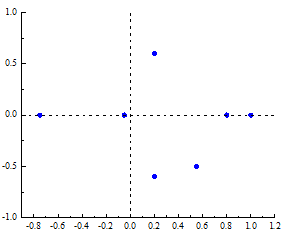(a) AR root graph of total energy consumption

## 4b.png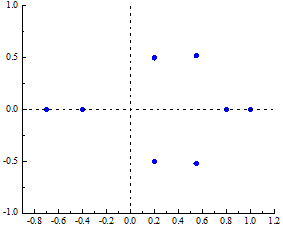(b) AR root graph of coal consumption

## 4c.png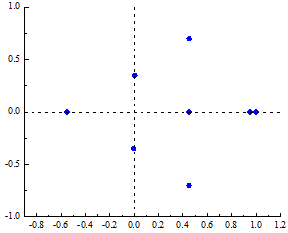(c) AR root graph of oil consumption

Figure 4. Results of the vector error correction model

3.2 Nonlinear dynamic relationship between energy consumption and economic growth

There are many nonlinear phenomena between China’s economic growth and energy consumption. In this paper, the GDP, total energy consumption, coal consumption and oil consumption in 2000~2018 are converted into logarithmic forms, and then subjected to correlation analysis. Figure 5 presents the residual distribution of nonlinear features. The nonlinear dynamic relationship between energy consumption and economic growth was tested by neural network. The results show that the variables of China’s energy consumption have certain nonlinear correlations with economic growth.

## 5.png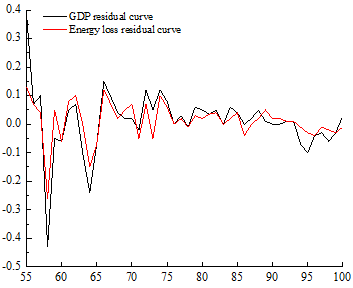Figure 5. The residual distribution of nonlinear features

4. Case Analysis

4.1 Thermoeconomic cost analysis on iron and steel enterprises

The steel industry is the main energy consumer and pillar of economic growth of China. The iron and steel enterprises mainly consume physical energy and chemical energy. The existing studies have shown that the blast furnace process consumes more energy than any other process, followed by rolling and refining.

For an iron and steel enterprise, considerable economic benefits can be obtained through rationalization of energy consumption. For example, steam, a byproduct of coke dry quenching (CDQ), can be used to reduce the cost of products like coke and coke oven gas (COG). In this way, the enterprise will receive more economic benefits at a low theormoeconomic cost. The utilization of steam only increases the cost of water, without pushing up the cost of any other energy. What is more, the extra stream is much more valuable than the water being consumed. Figure 6 lists the impacts of each process in the CDQ technology on the thermoeconomic cost of an iron and steel enterprise. The cost reductions of main products and byproducts in each process were clearly displayed. According to the figure, the CDQ technology can lower the coke cost by over RMB 14 million yuan/year if the enterprise produces tens of millions of cokes per year.

Coke is the main fuel in the blast furnace process. The cost of this process can be reduced by using the coke produced in the previous process, that is, recovering sinter sensible heat. Compared with the CDQ technology, the sinter sensible heat recovery (SSHR) further reduces the level and rate of energy consumption and enhances the output energy value, thus lowering the thermoeconomic cost. Figure 7 lists the impacts of each process in the SSHR on the thermoeconomic cost. The thermoeconomic cost can be suppressed markedly, because the steam recovered by the SSHR saves much more than the cost of water, and sinter is the main raw material of blast furnace process.

## 6.png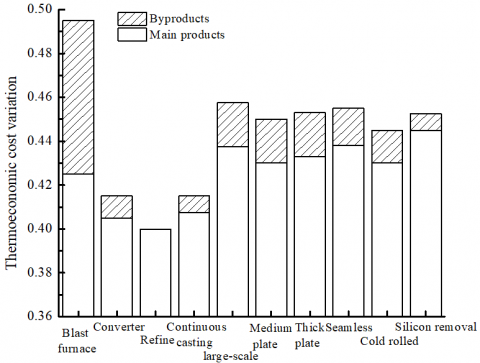Figure 6. The impacts of each process in the CDQ technology on the thermoeconomic cost

## 7.png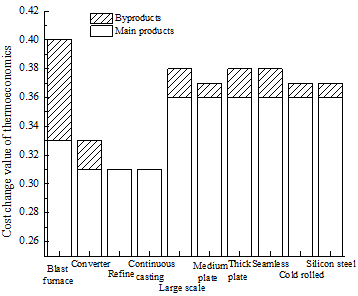Figure 7. The impacts of each process in the SSHR on the thermoeconomic cost

4.2 Thermoeconomic optimization based on genetic algorithm (GA)

The above analysis on energy consumption cost considers the energy cost of system operation, failing to address the investment, operation cost and maintenance cost of the system. To make up for the gap, the author analyzed the thermoeconomic performance of residual heat utilization system at three typical residual heat loads: 50 %, 75 % and 85 %. The residual heat utilization system was optimized by the GA, such that all main equipment, except the residual heat boiler and steam turbine, and the entire system consumed less energy. Figure 8 presents the cost variation of each subsystem per unit of energy. It can be seen that, with the growth in residual heat load, the cost of each subsystem per unit of energy increased first and then decreased, and minimized at the residual heat load of 75 %.

Figure 9 shows the variation in the mean cost of all subsystems per unit of energy with residual heat loads. Obviously, the mean cost of most subsystems had little to do with the residual heat load. The lowest cost per unit of energy was observed in Subsystem 12 at the residual heat load of 75 %. Overall, the GA optimization lowers the mean cost of all subsystems, and improves the thermoeconomic performance of the residual heat utilization system.

## 8.png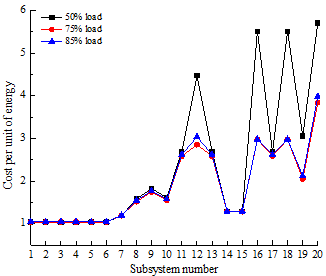Figure 8. The variation in the cost of each subsystem per unit of energy

## 9.pngFigure 9. The variation in variation in the mean cost of all subsystems per unit of energy

Table 1. Comparison between the performance parameters of the residual heat utilization system before and after the GA optimization

 Item Original value Optimized value Increment/Decrement Objective function 0.83803 0.81728 -2.48% Energy consumption 6737.09 6565.72 -2.54% Energy efficiency 56.04 57.01 1.73% Total generating capacity 673.34 820.42 21.84% Weighted generation cost 0.49405 0.42703 -13.57% Investment cost 3683601.9 3873288.8 5.15%

Table 1 compares the performance parameters of the residual heat utilization system before and after the GA optimization. The comparison shows that the GA optimization reduced the sum of external resource cost, total equipment investment and energy consumption by 2.48 %, lowered the energy consumption by 2.54 %, improved the overall energy efficiency by 1.73 %, increased the total generating capacity by 21.48 %, cut down the weighted generating cost by 13.57 % and boosted the investment cost by 5.15 %.

5. Conclusions

Based on thermoeconomic cost analysis model, this paper analyzes the relationship between energy consumption and economic growth in China, and verifies the analysis results through a case study on iron and steel enterprises. The main conclusions are as follows:

(1) The total energy consumption in China does not have a significant dynamic impact on economic growth, but economic growth exerts a significant impact on the total energy consumption; coal consumption and oil consumption have obvious dynamic effects on economic growth.

(2) The thermoeconomic cost analysis on an iron and steel enterprise shows that both the CDQ and the SSHR can greatly reduce the level and rate of energy consumption, and enhance the output energy value, thus lowering the thermoeconomic cost.

(3) With the growth in residual heat load, the cost of each subsystem per unit of energy increased first and then decreased, and minimized at the residual heat load of 75 %. Overall, the GA optimization lowers the mean cost of all subsystems, and improves the thermoeconomic performance of the residual heat utilization system.

References

 Ifaei, P., Ataei, A., Yoo, C.K. (2016). Thermoeconomic and environmental analyses of a low water consumption combined steam power plant and refrigeration chillers-part 2: Thermoeconomic and environmental analysis. Energy Conversion and Management, 123: 625-642. https://doi.org/10.1016/j.enconman.2016.06.030

 lukowicz, H., Dykas, S., Rulik, S., Stępczyńska, K. (2011). Thermodynamic and economic analysis of a 900 mw ultra-supercritical power unit. Archives of Thermodynamics, 32(3): 231-244. https://doi.org/10.2478/v10173-011-0025-1

 Wang, C., He, B., Yan, L., Pei, X., Chen, S. (2014). Thermodynamic analysis of a low-pressure economizer based waste heat recovery system for a coal-fired power plant. Energy, 65: 80-90. https://doi.org/10.1016/j.energy.2013.11.084

 Wang, M., Khalilpour, R., Abbas, A. (2014). Thermodynamic and economic optimization of LNG mixed refrigerant processes. Energy Conversion and Management, 88: 947-961. https://doi.org/10.1016/j.enconman.2014.09.007

 Aghbashlo, M., Rosen, M.A. (2018). Exergoeconoenvironmental analysis as a new concept for developing thermodynamically, economically, and environmentally sound energy conversion systems. Journal of Cleaner Production, 187: 190-204. https://doi.org/10.1016/j.jclepro.2018.03.214

 Kazemi, N., Samadi, F. (2016). Thermodynamic, economic and thermo-economic optimization of a new proposed organic Rankine cycle for energy production from geothermal resources. Energy Conversion and Management, 121: 391-401. https://doi.org/10.1016/j.enconman.2016.05.046

 Anjomshoaa, A., Salmanzadeh, M. (2018). Finding a criterion for the pressure loss of energy recovery exchangers in HVAC systems from thermodynamic and economic points of view. Energy and Buildings, 166: 426-437. https://doi.org/10.1016/j.enbuild.2018.02.016

 Villasmil, W., Steinfeld, A. (2010). Hydrogen production by hydrogen sulfide splitting using concentrated solar energy – thermodynamics and economic evaluation. Energy Conversion and Management, 51(11): 2353-2361. https://doi.org/10.1016/j.enconman.2010.04.009

 Chen, Hammond, G.P, Norman, J.B. (2016). Energy efficiency potentials: contrasting thermodynamic, technical and economic limits for organic Rankine cycles within UK industry. Applied Energy, 164: 984-990. https://doi.org/10.1016/j.apenergy.2015.06.046

 Heyne, S., Harvey, S. (2013). Assessment of the energy and economic performance of second generation biofuel production processes using energy market scenarios. Applied Energy, 101: 203-212. https://doi.org/10.1016/j.apenergy.2012.03.034

 Al-Mutairi, E.M., Odejobi, O.J. (2013). Investigating the thermodynamics and economics of operating the thermal power plant under uncertain conditions. Energy Conversion and Management, 75: 325-335. https://doi.org/10.1016/j.enconman.2013.06.029

 Tan, M., Kecebas, A. (2014). Thermodynamic and economic evaluations of a geothermal district heating system using advanced exergy-based methods. Energy Conversion and Management, 77: 504-513. https://doi.org/10.1016/j.enconman.2013.10.006

 Maslov, V.P. (2009). Mathematical economics and thermodynamics: crises as phase transitions. Mathematical Notes, 86(5-6): 879-882. https://doi.org/10.1134/S0001434609110303

 Yilmaz, C. (2018). Thermoeconomic cost analysis and comparison of methodologies for Dora ii binary geothermal power plant. Geothermics, 75: 48-57. https://doi.org/10.1016/j.geothermics.2018.04.002